Wavelength modulation spectroscopy at 1530.32 nm for measurements of acetylene based on Fabry–Perot tunable filter
School of Physics and Electronics, Central South University, Changsha 410083, China

† Corresponding author. E-mail: bingchuyang@csu.edu.cn

‡ Corresponding author. E-mail: xuxuemei999@126.com

Project supported by the National Natural Science Foundation of China (Grant Nos. 61172047 and 61071025).

Abstract
Abstract

Sensitive detection of acetylene (C2H2) is performed by absorption spectroscopy and wavelength modulation spectroscopy (WMS) based on Fiber Fabry–Perot tunable filter (FFP-TF) at 1530.32 nm. After being calibrated by Fiber Bragg Grating (FBG), FFP-TF is frequency-multiplexed and modulated at 20 Hz and 2.5 kHz respectively to achieve wavelength modulation. The linearity with 0.9907 fitting coefficient is obtained by measuring different concentrations in a 100 ppmv–400 ppmv range. Furthermore, the stability of the system is analyzed by detecting 50 ppmv and 100 ppmv standard gases for 2 h under room temperature and ambient pressure conditions respectively. The precision of 11 ppmv is achieved by calculating the standard deviation. Therefore, the measuring system of C2H2 detection can be applied in practical applications.

1. Introduction

C2H2 is a species of toxic gases that have strong chemical activities, and it is easy for them to decompose, burn, and explode. In the field of transformer fault diagnosis, C2H2 is one of the main components of the oil-dissolved fault gases in the transformer. Besides, during the research of plant growth, C2H2 can be used as a kind of plant hormone to ripen the fruits or wither the flowers. Therefore, sensitive C2H2 detection is extremely significant in some practical applications.

For now, there are two major methods of detecting C2H2. One is gas chromatography, which is very universal and valid in industrial applications. However, the method is process-complicated and time-consuming. Hence, it is not suitable for on-line monitoring. The other is tunable diode laser absorption spectroscopy (TDLAS). After selecting the apposite absorption line of the gas to be measured, it is easy to achieve highly sensitive and good selective results by scanning a single narrow laser line around the chosen absorption line. To improve gas optical absorption length and measurement sensitivity, a multi-pass absorption cell was proposed. Furthermore, with the development of wavelength modulation technology, the second harmonic detection method was employed in measuring the densities to obtain higher signal-to-noise ratio (SNR). Various trace gases have been detected successfully by this method.

In some special applications, it is very necessary to measure various gases at the same time. For example, during transformer fault diagnosis, it is usually required to detect several oil-dissolved fault gases simultaneously, such as acetylene (C2H2), ethylene (C2H4), methane (CH4), etc. A logical solution of TDLAS is to use multiple distributed feedback (DFB) lasers corresponding to each target gas. Therefore, with the increase of gas species, the gas detection system will become complicated and confused. In the present paper, the DFB laser is substituted by an amplified spontaneous emission (ASE) broadband optical source to detect C2H2 concentration. FFP-TF[10,11] is used to achieve wavelength modulation, and the Fiber Bragg Grating (FBG) is applied to the calibration of FFP-TF. Because the FFP-TF has a broad tunable spectral range, the system hardly requires any change while sensing multi-component gas. In addition, according to the Pacific Northwest National Laboratory (PNNL) spectra database and spectral data from experiment, the apposite absorption line of C2H2 is determined. Moreover, the linearity of the system is tested by measuring different C2H2 concentrations. Besides, the background signals of system due to the fluctuation of ASE source and electric noise are also considered. Finally, the precision and the stability of the gas measurement system are analyzed by the standard deviation.[13,14]

2. Design of system
2.1. Theoretical principles

The fundamentals of absorption spectroscopy, wavelength modulation and FFP-TF are presented to guide readers in understanding the system and the discussion presented in Section 3. The absorption of monochromatic light along a uniform path can be described by the Beer–Lambert law,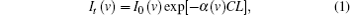where It (v) and I0 (v) are the transmitted and incident light intensities at optical frequency v (cm−1), α(v) (L·g−1·cm−1) is the absorption coefficient which is related to line-strength and line-shape function at optical frequency v, C (g·L−1) is the gas concentration, and L (cm) is the path length.

To improve SNR, wavelength modulation is introduced into the system. In the present paper, the injection voltage of FFP-TF is modulated by a sinusoidal function at frequency f, resulting in simultaneous modulation of passed wavelength (or frequency),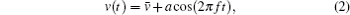where (cm−1) is the center wavelength (or frequency) of the passed wavelength of FFP-TF without modulation, and a (cm−1) is the modulation depth. Because the value of a is small enough, the incident light intensity can be considered to remain unchanged, that is,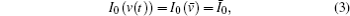where Ī0 is the incident light intensity without modulation. Under the condition of the weak absorption of gases, the Beer–Lambert relation with modulation can be described by the following equation: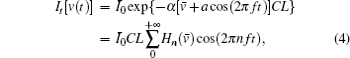where Hn() is the n-th order Fourier coefficient. According to the existing literature,[15,16] the value of odd harmonics at is always zero, however, the value of even harmonics at is always maximal, and it becomes smaller with the increase of harmonic number, which indicates that the second harmonic value is maximal. Therefore, the gas concentration can be calculated by measuring the amplitude of the second harmonic signal.

The basic theory of FFP-TF is the multi-beam interference principle. The input and output fibers are fastened to a pair of piezoelectric ceramics, that is, F-P cavity length can be controlled by the operating voltage applied to the pair of piezoelectric ceramics. According to the F-P interference principle, if the F-P cavity length alters, the center wavelength of narrowband interference peak changes, and then the transmission wavelength of FFP-TF shifts. Figures 1(a) and 1(b) show the different transmission wavelengths of FFP-TF with positive and negative scan voltage which work on the FFP-TF respectively. It can be seen that transmission wavelength and scan voltage each have a good linear relation with 0.999 fitting coefficient. Therefore, wavelength modulation can be implemented by controlling the external operating voltage applied to FFP-TF.

 Figure Option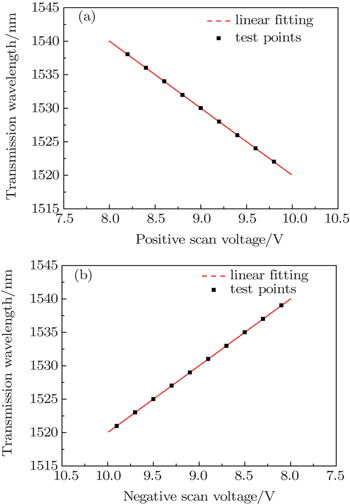Fig. 1. Transmission wavelengths versus positive scan voltage (a) and negative scan voltage (b).
2.2. Setup of wavelength modulation spectroscopy system

On the basis of theories just described above, the WMS system based on FFP-TF is designed as shown in Fig. 2. In the system, ASE broadband light source covers C Band (1520 nm–1570 nm) which contains C2H2 gas absorption spectrum within the near-infrared region. FFP-TF with a magnitude of about 0.003 nm/mV is employed to filter the broadband light from ASE for generating narrowband light whose 3-dB bandwidth is 10 pm. The scan voltage of FFP-TF is provided by a signal generator that is controlled by a personal computer (PC) for signal processing. The signal from the signal generator contains not only a triangular wave of 20 Hz which linearly sweeps over a wavelength of 1530.32 nm where C2H2 has the strongest absorption, but also a 2.5-kHz sine wave which modulates the transmission wavelength of FFP-TF. In addition, a 1-in-2 (90:10) coupler is used to divide the narrowband light from FFP-TF into two parts. One passes through the gas cell and then it is detected by InGaAs photodetector1 (PD1), and in this case the electric signal from PD1 is sent to the lock-in amplifier for demodulation. At the same time, the 5-kHz sine wave (to detect the second harmonic signal) from the signal generator is also sent to the lock-in amplifier. The second harmonic signal from the lock-in amplifier is directed to a data acquisition (DAQ) unit, and finally reaches the PC for signal processing to determine the C2H2 concentration.

 Figure Option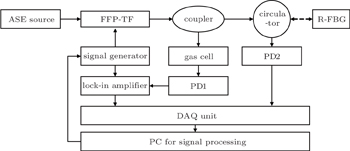Fig. 2. Schematic diagram of the experiment setup for WMS system.

The other part from the coupler is used to calibrate FFP-TF. The FFP-TF is sensitive to temperature because of its construction, that is, when the ambient temperature changes, the transmission wavelength of FFP-TF will shift as shown in Fig. 3, and it is noticeable that the driving voltage of FFP-TF remains the same in the experiment. This will have adverse effects on wavelength modulation. Therefore, a reference fiber Bragg grating (R-FBG) that can reflect a particular wavelength of light and transmit all others, is employed. In the system, an R-FBG with reflective center wavelength of 1530 nm is chosen. Because FBG is sensitive to both strain and temperature, to obtain highly stable FBG, the strain and temperature acting on the FBG need to remain unchanged. In the measurement process, the R-FBG does not bear the external strain. Consequently, a thermostatic apparatus is used to ensure the stability of R-FBG.

 Figure Option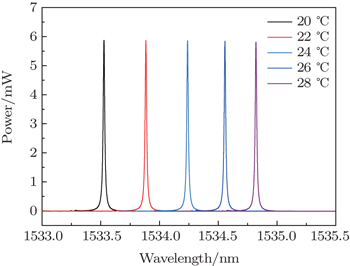Fig. 3. Powers versus transmission wavelength of FFP-TF for different temperatures.

The narrowband light from the coupler passes through a circulator and reaches the R-FBG, and then is reflected (only a specific range of wavelengths can be reflected) by R-FBG. The light reflected by R-FBG comes back to the circulator and is detected by InGaAs photodetector2 (PD2). The electric signal from PD2 is sent to the DAQ unit and eventually reaches the PC for signal processing. By analyzing the signal from PD2, the relation between driving voltage of FFP-TF and reflective central wavelength of R-FBG can be calculated. Then the PC for signal processing controls the signal generator to adjust the scan voltage of FFP-TF appropriately for achieving wavelength modulation. This approach can calibrate FFP-TF effectively and eliminate the influence of ambient temperature.

3. C2H2 concentration measurement and discussion
3.1. Selection of C2H2 absorption line

When choosing the absorption line of C2H2, there are two problems to be considered. One is about the spectral absorption line interference from C2H2 itself or other gases. The other is about the intensity of C2H2 absorption line, which determines the detection limit and precision of the system. That is, the sensitivity is higher with greater intensity. Figure 4(a) shows the C2H2 absorption lines in a range from 1525 nm to 1545 nm from PNNL spectroscopic database at 296 K, and figure 4(b) shows the C2H2 absorption lines from the experiment at room temperature and ambient pressure in the same spectral region. In the experiment, since the power of laser does not stay unchanged as shown in Fig. 4(b), there are some small differences between Figs. 4(a) and 4(b). However, by comparison, it is found that the numbers and the positions of absorption lines in Figs. 4(a) and 4(b) are the same. In addition, the trend of absorption line intensity is also analogous. To obtain high sensitivity and SNR, the absorption line with a center wavelength of 1530.32 nm that has the strongest intensity in the near-infrared region is chosen, which is shown in the rectangular box in Figs. 4(a) and also in Fig. 4(b).

 Figure Option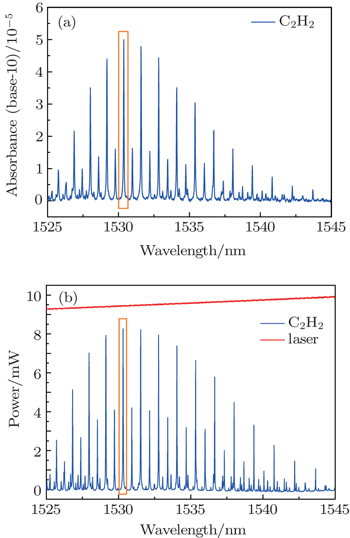Fig. 4. C2H2 absorption lines from PNNL database (a) and experiment (b).
3.2. System linearity

The initial second harmonic (2f) signal in Fig. 5 is demodulated from WMS raw signal at 5 kHz. The demodulated 2f signal shows an additive background caused by residual amplitude modulation (RAM) due to the minor fluctuations of ASE source at different wavelengths and electronic noises. To correct this background, measurements without the C2H2 absorption are conducted. A series of the background measurements is taken to obtain a stable RAM background in Fig. 5. The corrected 2f signal that is obtained by subtracting the stable background is shown in Fig. 5. Obviously, the symmetry and accuracy of the 2f signal are considerably improved after background correction.

 Figure Option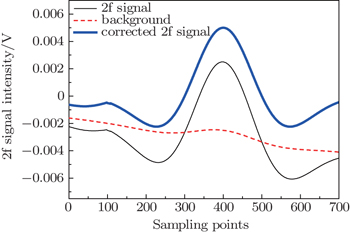Fig. 5. The 2f signals before and after background subtraction.

Linearity is one important parameter in a measuring instrument. In the system, standard gases with C2H2 concentrations of 100 ppmv, 200 ppmv, 300 ppmv, and 400 ppmv are detected successively to verify the linearity. Figure 6 shows the corrected 2f signals for different concentrations. Furthermore, the concentrations and the peak-to-peak values are linearly fitted in Fig. 7. The result shows that the system has a good linearity with 0.9907 fitting coefficient, which is the basis of accurate measurement.

 Figure Option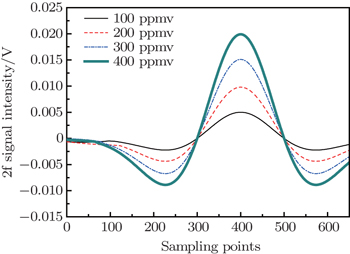Fig. 6. Corrected 2f signals versus sampling point for different concentrations.
 Figure Option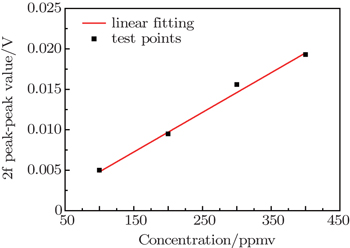Fig. 7. Linearity of the measurement system. The fitting is given by y = 0.0001(0.49x − 1) with 0.9907 fitting coefficient.
3.3. System stability

Standard deviation is usually used to measure the stability of the instrument performance, that is, the better stability gives rise to the less standard deviation. In the analysis experiment, standard gases of 50-ppmv C2H2 and 100-ppmv C2H2 are tested for a time of 2 h respectively as shown in Fig. 8. The standard deviations of 50 ppmv and 100 ppmv are 1.99 ppmv and 1.89 ppmv respectively. Therefore, the system has a good stability because the standard deviations are small and similar. Besides, the resulting ranges of fluctuations are 10.85 ppmv and 11.06 ppmv respectively, which indicates that the precision of the system is around 11 ppmv. Moreover, the measurement results are analyzed by calculating the probability distribution as shown in Fig. 9, which shows that the measurement noises are clearly Gaussian distributed.

 Figure Option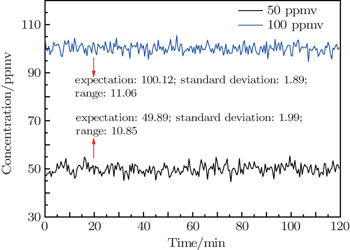Fig. 8. Measurements of 50-ppmv and 100-ppmv C2H2 standard gases.
 Figure Option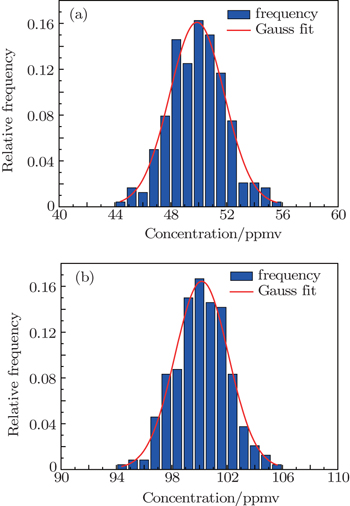Fig. 9. Probability distributions of measurements for 50 ppmv (a) and 100 ppmv (b).
4. Conclusions

Wavelength modulation spectrum at 1530.32 nm for the measurement of C2H2 based on FFP-TF is presented. Instead of traditional tunable diode laser, ASE broadband source and FFP-TF are used to achieve wavelength modulation. Under room temperature and ambient pressure conditions, a number of experiments are performed with this system. The experimental results indicate that the system has a good linearity and stability. Although the precision of the system is only 11 ppmv, it still meets the requirements of some practical applications and offers the possibility of measuring species of gases simultaneously without additional installation.

Reference
 1 Wang M HHung C P 2003 Electric Power Syst. Res. 67 53 2 Fattahi NAssadi YHosseini M R MJahromi E Z 2007 Chromatogr. A 1157 23 3 Werle PMücke RSlemr F 1993 Appl. Phys. B 57 131 4 Lackner M 2007 Rev. Chem. Eng. 23 65 5 Hunsmann SWunderle KWagner SRascher USchurr UEbert V 2008 Appl. Phys. B 92 393 6 Liu J T CJeffries J BHanson R K 2004 Appl. Phys. B 78 503 7 Chao XJeffries J BHanson R K 2012 Appl. Phys. B 106 987 8 Xia HDong F ZWu BZhang Z RPang TSun P SCui X JHan LWang Y 2015 Chin. Phys. B 24 034204 9 Werle P 2011 Appl. Phys. B 102 313 10 Bao YSneh AHsu KJohnson K MLiu J YMiller C MMonta YMcClain M B 1996 IEEE Photon. Technol. Lett. 8 1190 11 Han Y GDong XKim C SJeong M YLee J H 2007 Opt. Express 15 2921 12 Jun CVilliger MOh W YBouma B E 2014 Opt. Express 22 25805 13 Sun KChao XSur RJeffries J BHanson R K 2013 Appl. Phys. B 110 497 14 Klein AWitzel OEbert V 2014 Sensors 14 21497 15 Goldenstein C SSpearrin R MSchultz I AJeffries J BHanson R K 2014 Measur. Sci. Technol. 25 055101 16 Lu CDing Y JPeng Z MLi X H 2012 Chin. Phys. B 21 127803